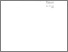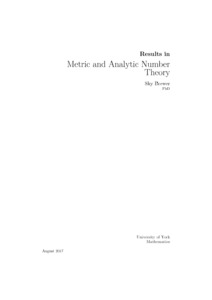# Results in Metric and Analytic Number Theory

Brewer, Sky J (2017) Results in Metric and Analytic Number Theory. PhD thesis, University of York.Preview
Text
Final Version Thesis - Sky Brewer.pdf - Examined Thesis (PDF)
The following Thesis consists of five chapters. The first three chapters come from Metric Number theory. Chapter 1 discusses algorithms for calculating the continued fraction of algebraic numbers, as well as presenting experimental results on how well algebraic numbers fit well known conjectures on the distribution of their partial quotients. Chapter 2 discusses the Singular and Extremality theories of so called well separated Dirichlet type systems''. Chapter 3 presents an effective version of the Khintchine-Groshev theorem for simultaneously small linear forms. The last two chapters are mostly in the area of uniform distribution. Chapter 4 proves a central limit theorem for the count of the fractional parts of imaginary parts of the zeros of the Riemann zeta function within an interval. Chapter 5 discusses the upper and lower distribution functions mod $1$ of sequences of the form $(0.a_na_{n+1}a_{n+2} \dots)_{n \in\mathbb N}$, where the sequence $(a_n)_{n \in \mathbb N}$ has polynomial growth.